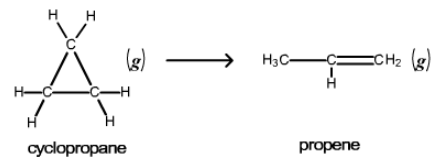# Problem: Consider the first-order reaction described by the equation cyclopropane propeneAt a certain temperature, the rate constant for this reaction is 5.62x10-4 s-1. Calculate the half-life of cyclopropane at this temperature. Given an initial cyclopropane concentration of 0.00850 M, calculate the concentration of cyclopropane that remains after 2.20 hours.

###### FREE Expert Solution

Part A

We are being asked to determine the half-life of the following reaction

cyclopropene, C3H6 (g) → propene, C3H6 (g)

We will use the following formula to solve this problem

90% (159 ratings)###### Problem Details

Consider the first-order reaction described by the equation cyclopropane propeneAt a certain temperature, the rate constant for this reaction is 5.62x10-4 s-1. Calculate the half-life of cyclopropane at this temperature.

Given an initial cyclopropane concentration of 0.00850 M, calculate the concentration of cyclopropane that remains after 2.20 hours.